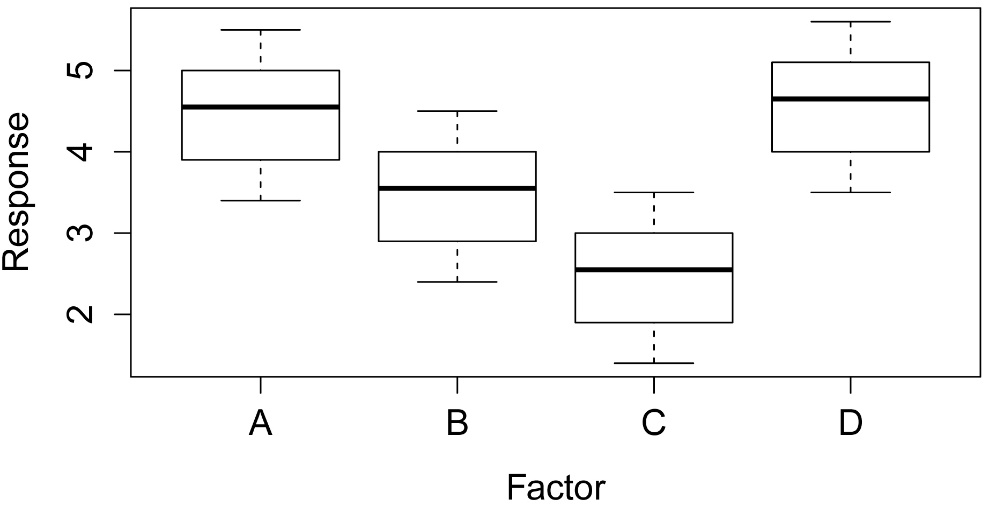## An R Companion for the Handbook of Biological Statistics

Salvatore S. Mangiafico

# One-way Analysis with Permutation Test

Permutation tests are non-parametric tests that do not assume normally-distributed errors.  However, these tests may assume that distributions have similar variance or shape to be interpreted as a test of means.

A one-way anova using permutation tests can be performed with the coin package.  A post-hoc analysis can be conducted with pairwise permutation tests analagous to pairwise t-tests.  This can be accomplished with the functions pairwisePermutationTest and pairwisePermutationMatrix in the rcompanion package, which rely on the  independence_test function in the coin package.

For more information on permutation tests available in the coin package, see:

help(package="coin")

Consult the chapters on One-way Anova and Kruskal–Wallis Test for general consideration about conducting analysis of variance.

### Packages used in this chapter

The following commands will install these packages if they are not already installed:

if(!require(coin)){install.packages("coin")}
if(!require(FSA)){install.packages("FSA")}
if(!require(rcompanion)){install.packages("rcompanion")}
if(!require(multcompView)){install.packages("multcompView")}

#### Permutation test for one-way analysis

### --------------------------------------------------------------
### One-way permutation test, hypothetical data

### --------------------------------------------------------------

Input =("
Factor Response
A     4.6
A     5.5
A     3.4
A     5.0
A     3.9
A     4.5
B     3.6
B     4.5
B     2.4
B     4.0
B     2.9
B     3.5
C     2.6
C     3.5
C     1.4
C     3.0
C     1.9
C     2.5
D     4.7
D     5.6
D     3.5
D     5.1
D     4.0
D     4.6
")

Data\$Factor = factor(Data\$Factor,
ordered=FALSE,
levels=unique(Data\$Factor))

# Order factors, otherwise R will alphabetize them

boxplot(Response ~ Factor,
data = Data,
ylab ="Response",
xlab ="Factor")##### Permutation test

library(coin)

independence_test(Response ~ Factor,
data = Data)

Asymptotic General Independence Test

maxT = 3.2251, p-value = 0.005183

#### Pairwise permutation tests

Pairwise permutation tests could be used as a post-hoc test for a significant permutation test.  If no p-value adjustment is made, then the type I error rate may be inflated due to multiple comparisons.  Here, the “fdr” p-value adjustment method is used to control the false discovery rate.

##### Table output with pairwisePermutationTest

### Order groups by median

Data\$Factor = factor(Data\$Factor,
levels = c("D", "A", "B", "C"))

library(FSA)

### Pairwise tests

library(rcompanion)

PT = pairwisePermutationTest(Response ~ Factor,
data = Data,
method="fdr")

PT

1  D - A = 0 -0.2409   0.8096  0.80960
2  D - B = 0  -2.074  0.03812  0.06106
3  D - C = 0  -2.776 0.005505  0.01876
4  A - B = 0   1.952  0.05088  0.06106
5  A - C = 0   2.734 0.006253  0.01876
6  B - C = 0   1.952  0.05088  0.06106

library(rcompanion)

data = PT,
threshold  = 0.05)

Group Letter MonoLetter
1     D      a         a
2     A      a         a
3     B     ab         ab
4     C      b          b

##### Compact letter display output with pairwisePermutationMatrix

### Order groups by median

Data\$Factor = factor(Data\$Factor,
levels = c("D", "A", "B", "C"))

library(FSA)

### Pairwise tests

library(rcompanion)

PM = pairwisePermutationMatrix(Response ~ Factor,
data = Data,
method="fdr")

PM

D      A       B        C
D NA 0.8096 0.03812 0.005505
A NA     NA 0.05088 0.006253
B NA     NA      NA 0.050880
C NA     NA      NA       NA

\$Method
 "fdr"

D       A       B       C
D 1.00000 0.80960 0.06106 0.01876
A 0.80960 1.00000 0.06106 0.01876
B 0.06106 0.06106 1.00000 0.06106
C 0.01876 0.01876 0.06106 1.00000

library(multcompView)